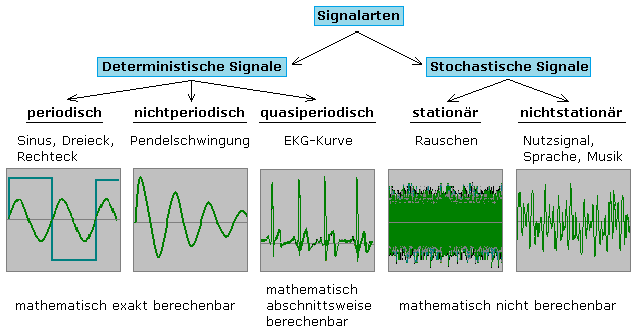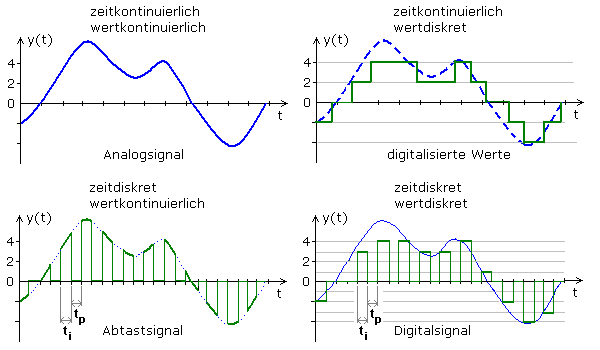# What are the types of signals

## Characterization of electrical signals

In communication technology, messages and data for forwarding and processing are encrypted in certain signals. DIN 44300 defines signals as physical carriers for data. The smallest unit of information is the sign. Data is a string of specific information that becomes a message when it is transmitted. An electrical signal is the representation of a message using physical quantities such as voltage, current, frequency, phase position, magnetic field strength. The signals for message transmission can almost always be described by time functions. The information is encoded in the change in one or more physical quantities.Deterministic signals can be described using functions. Their function values ​​are determined by mathematical expressions and arithmetic sequences and are thus determined. The amplitude values ​​of stochastic signals are random and therefore cannot be calculated exactly. A description can only be made according to mathematical statistical rules. Often a mean value or an autocorrelation function can be determined. It is the time average of the product of a function f (t) with the same function f (t + τ) shifted by the time τ.

Useful signals, such as speech, music and image signals, are stochastic signals with meaningful information content. Noise and other interfering signals are also stochastic and almost always part of every signal. As long as the signals perceived as disruptive factors remain negligibly small, efforts are made to replace stochastic signals with idealized, deterministic signals, since they are easier to describe mathematically.

The most important signal parameters associated with the information are the amplitude, the linear and root mean square value, the rms value, the frequency, the duty cycle, the phase position, the variance of the noise amplitude and the spectral composition of a signal. Both the value and the temporal change in the information-carrying signal variable can take place continuously or discontinuously, discretely. This results in four combinations that have their own names:In the case of time-discrete signals, the function value in the key pauses is not zero. It is not defined there. The conversion of an analog signal into a time-continuous, value-discrete signal is also referred to as quantization. The reverse of the process or reverse conversion is called smoothing and cannot be done without errors. If the two signals can be compared with one another, the error signal can be represented as quantization noise. The conversion of an analog signal into a sampling signal is called sampling. The reverse conversion is called interpolation and can be carried out relatively error-free with a small step size.

Analog signal

The information-carrying quantity of the analog signal is continuous in both value and time. The information is contained in the transmitted amplitude value. Information and signal quantities are clearly and reversibly linked to one another.

Digitized values

The information-carrying size of digitized values ​​is discrete in value but continuous over time. The sampling time is arbitrary and not fixed. The term digital here only describes constant amplitude jumps. Intermediate signal values ​​are not recorded at the moment of sampling.

Scanning signal

The information-carrying size of the scanning signal is value-continuous but time-discrete. The measured values ​​are recorded at a fixed time cycle. The amplitude value changes continuously within the pulse duration.

Digital signal

The information-carrying size of the digital signal is both discrete in value and discrete in time. A symmetrical, equidistant time cycle is often used. These digital signals can then be further coded into binary signals. The signal sequence then consists of high and low states.

Finally, the most important properties of electrical signals in a summary:

Analog signals are always time and value continuous.
Periodic signals can be represented using Fourier series.
Digital signals are always time and value discrete.
Determined signals:
The signal sizes are clearly reproducible. The signal course is known for all points in time.
Stochastic signals:
The signal sizes are not clearly reproducible. The signal course can be described by statistical properties.